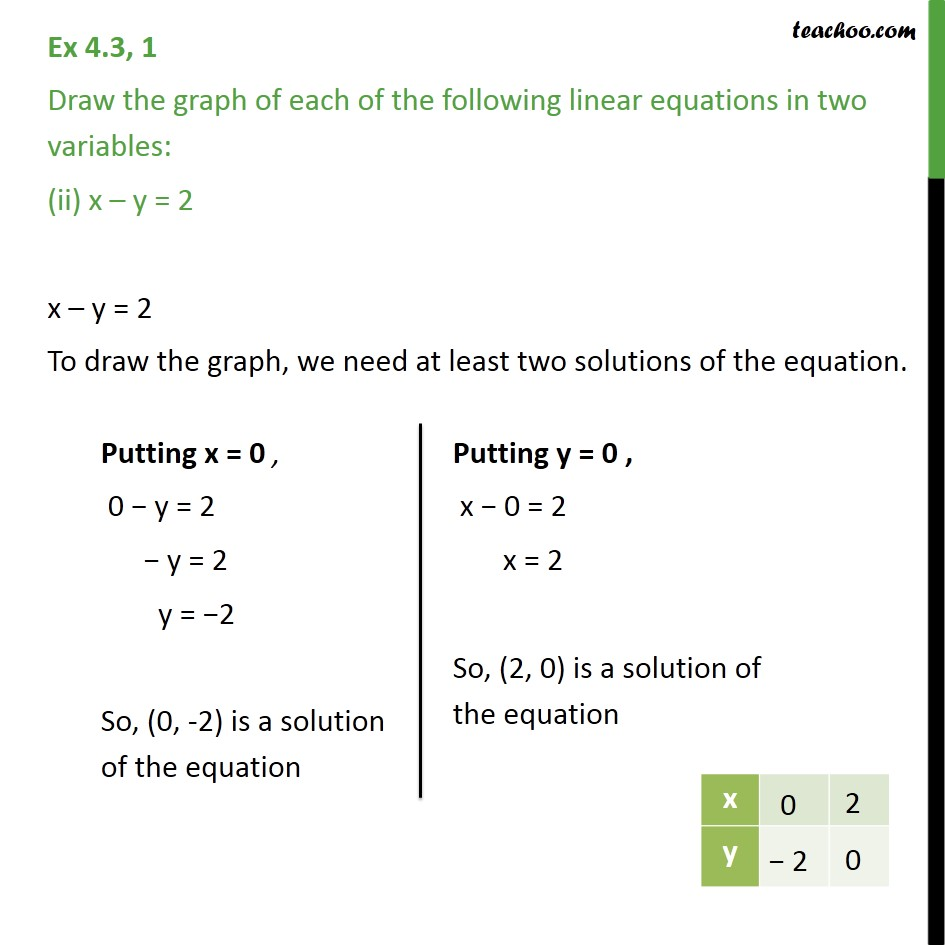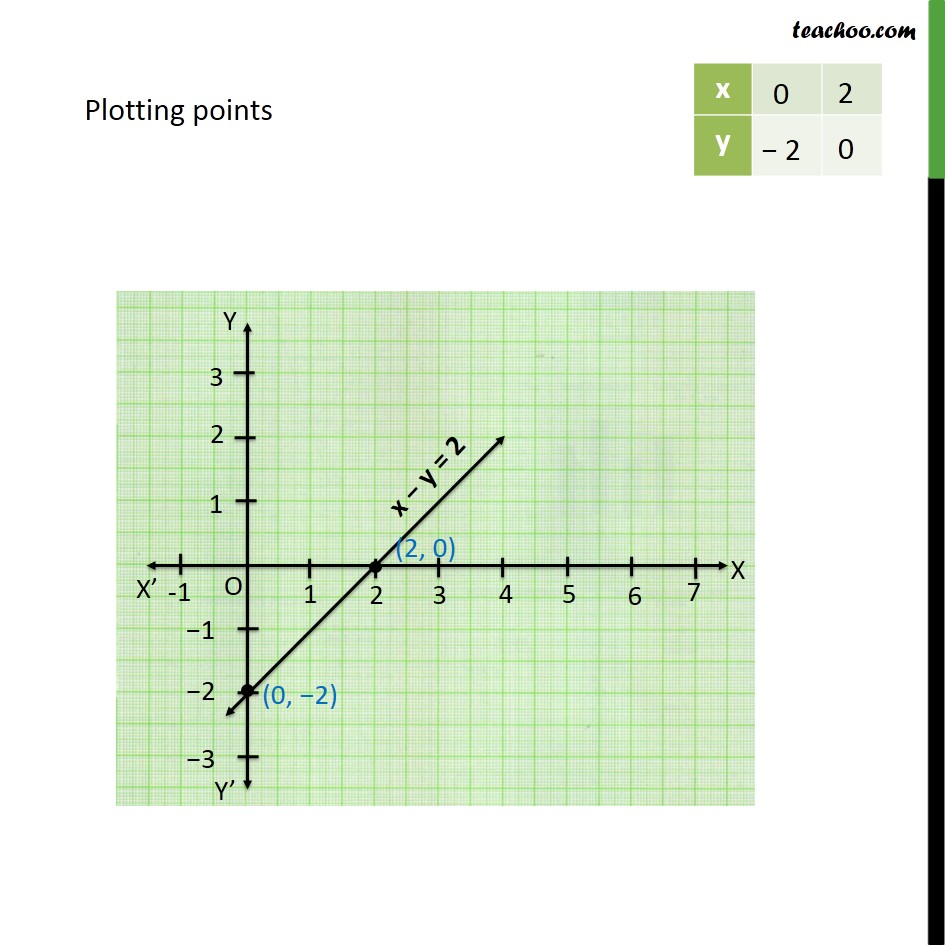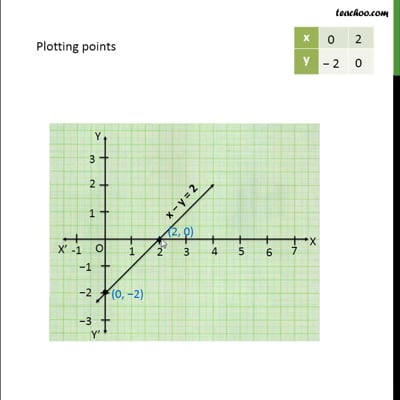Graph of Linear Equations

Chapter 4 Class 9 Linear Equations in Two Variables
Serial order wiseThis video is only available for Teachoo black users

Learn in your speed, with individual attention - Teachoo Maths 1-on-1 Class

### Transcript

Question 1 Draw the graph of each of the following linear equations in two variables: (ii) x – y = 2 x – y = 2 To draw the graph, we need at least two solutions of the equation.